## What is the direction of the force exerted on this particle by a magnetic field with magnitude 2.00 TT in the +z+z direction? Express your a

Question

What is the direction of the force exerted on this particle by a magnetic field with magnitude 2.00 TT in the +z+z direction? Express your answer in degrees measured counterclockwise from the +y+y axis.

in progress 0
6 months 2021-08-11T00:21:57+00:00 1 Answers 2 views 0

145 degree

Explanation:

We are given that

Magnetic field=B=2 T

Charge on particle,q=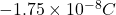Velocity, v=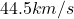Angle,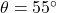We have to find the direction of the force  exerted on this particle by magnetic field with magnitude 2 T in the z direction.

Component of velocity along x- axis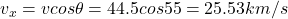Along y- axis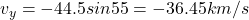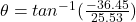Hence, the direction of force =145 degree